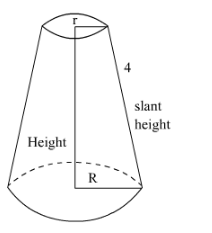# The slant height of the frustum of a cone is 4 cm`
Question:

The slant height of the frustum of a cone is 4 cm and the circumferences of its circular ends are 18 cm and 6 cm. Find curved surface area of the frustum.

Solution:

It is given that slant height of frustum of a cone is 4 cm. Circumferences of its ends are

18 cm and 6 cm.

We have to find the curved surface area of the frustum.Let l be the slant height

Letbe the radii of two circular ends of the cone

Circumference of one endCircumference of other endNow,

$2 \pi r=6$

$\pi r=3$

$2 \pi R=18$

$\pi R=9$

$\pi R+\pi r=9+3 \pi R+\pi r=9+3$

$\pi(R+r)=12 \quad \pi(R+r)=12$

Curved surface area of frustum $=\pi(r+R) l$

$=12 \times 4($ since $\pi(R+r)=12)$

$=48 \mathrm{~cm}^{2}$